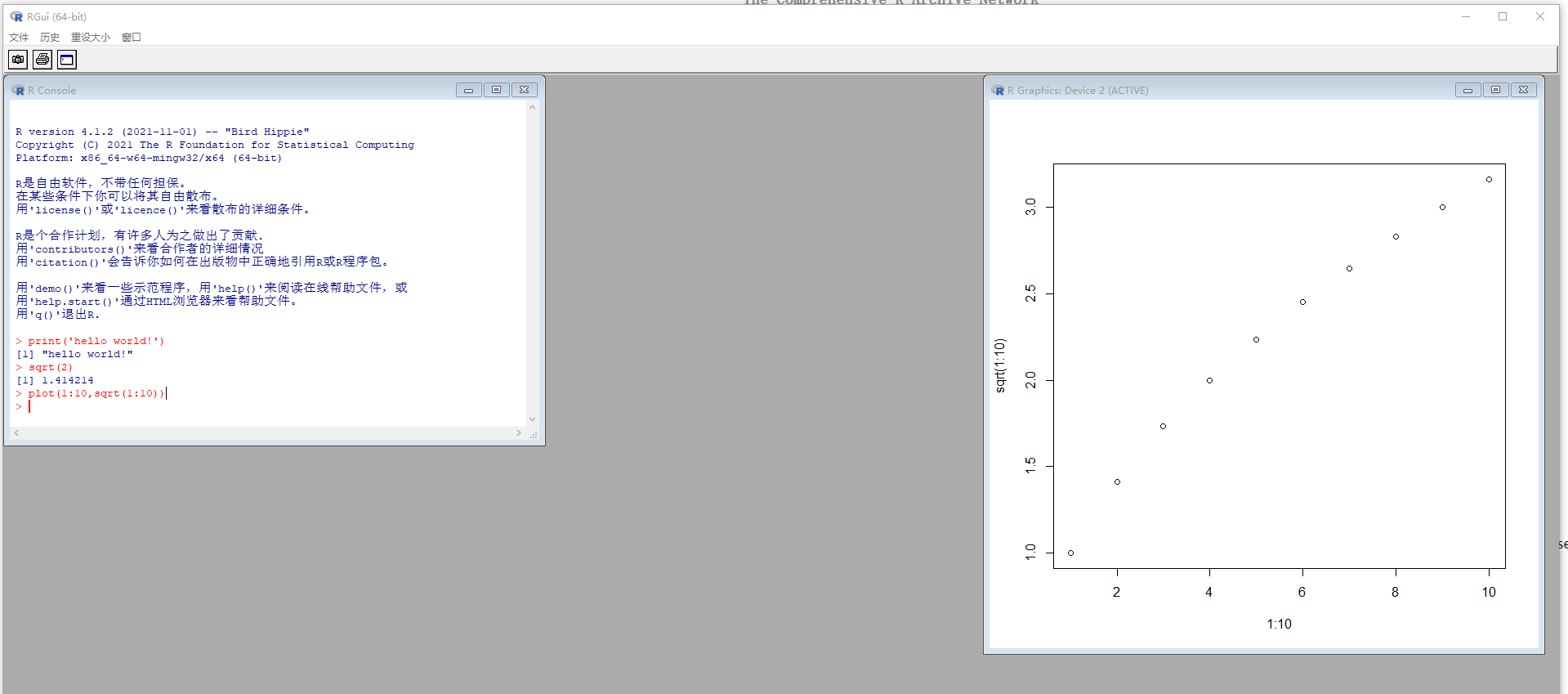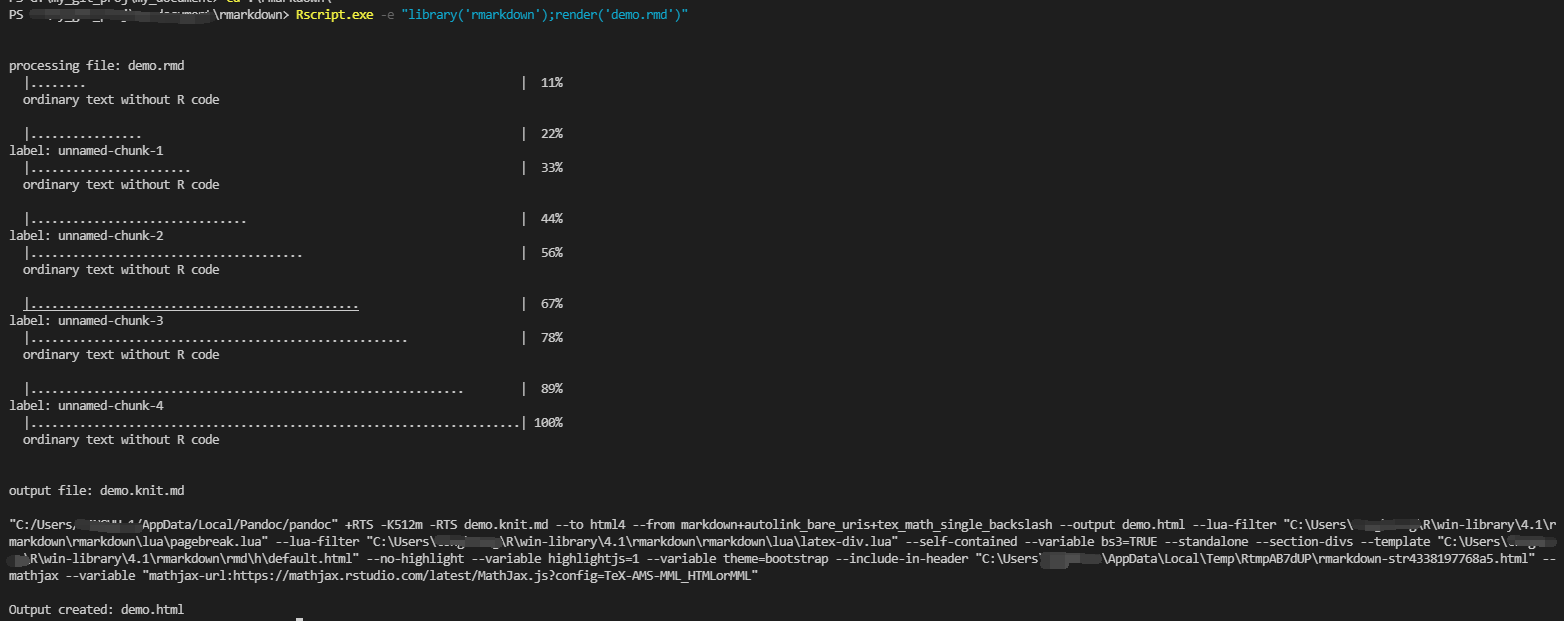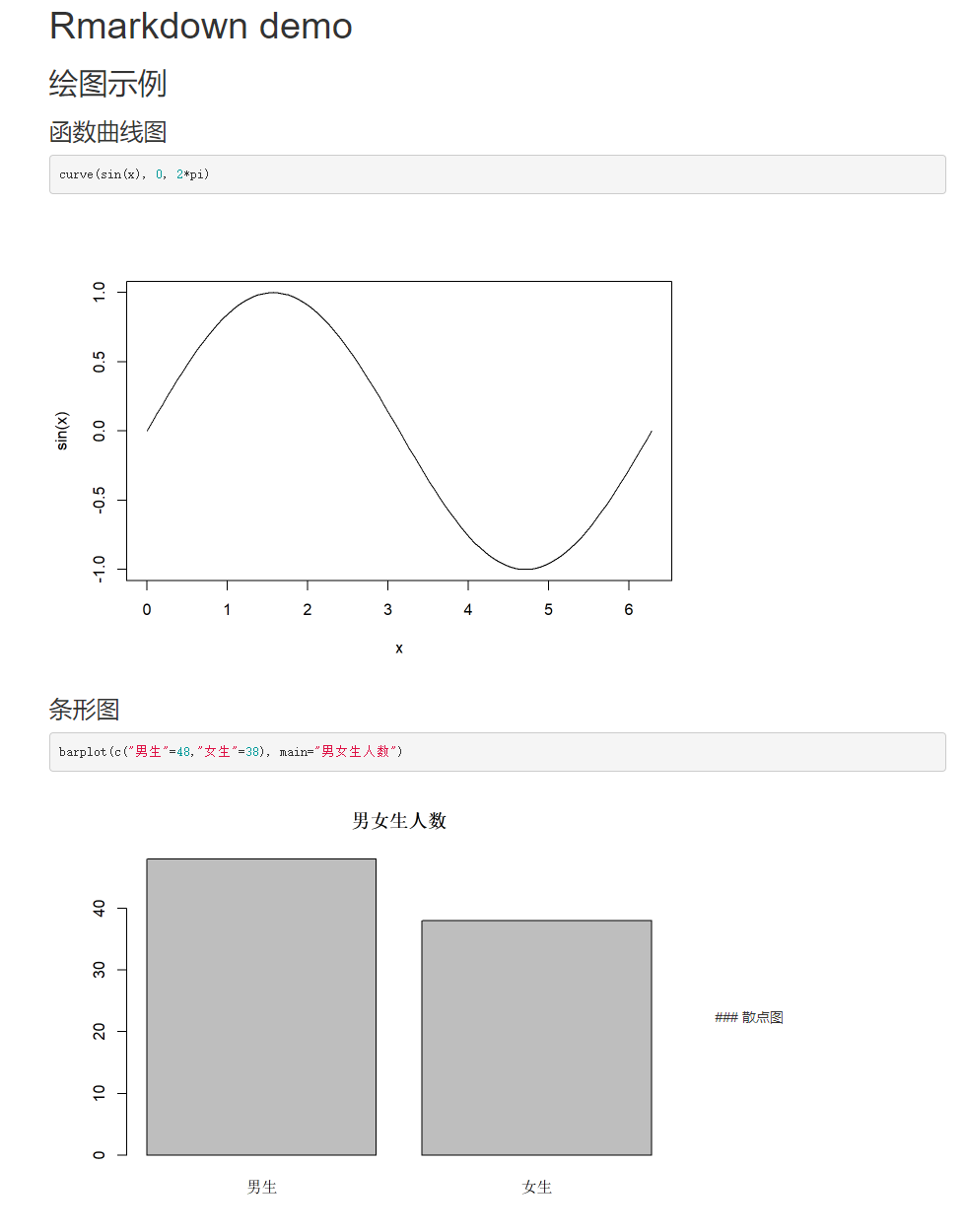## R 基础实战

• 安装比较简单，从官网下载后执行，可以只选择 x64；

• 使用R Gui执行 R 命令：

• 运行R Gui

• 尝试执行命令：

print('hello world!')

sqrt(2)
 1.414214
> plot(1:10,sqrt(1:10))

• 看下执行效果：• 使用命令行执行：

• 设置环境变量，将C:\Program Files\R\R-4.1.2\bin\x64添加到PATH中；
• 命令行指定语句
• 执行rscript.exe -e "sqrt(1:10)"，可以看到命令行能直接输出
• 执行rscript.exe -e "plot(1:10,sqrt(1:10))"，这时候看不到窗口输出，在当面目录会生成Rplots.pdf文件；
• 执行文件
• 执行rscript.exe -f "demo.r"
• 使用命令行执行指定文件：

• 使用任意编辑器创建文件demo.r，里面内容如下

print("hello world!")
sqrt(1:10)

• 执行rscript.exe "demo.r"

## R markdown 实战

### 编写 Rmarkdown 文件

---
title: "Rmarkdown demo"
output: html_document
---

## 绘图示例

### 函数曲线图

{r}
curve(sin(x), 0, 2*pi)


### 条形图

{r}
barplot(c("男生"=48,"女生"=38), main="男女生人数")


### 散点图

{r}
plot(1:100, sqrt(1:100))


## 汇总统计

{r}
knitr::kable(table(data[['征收方式']]))
knitr::kable((table(data[['征收方式']], data[['申报渠道']])))
summary(data[['营业额']])



### 编译 Rmarkdown 文件### 查看 html 文件